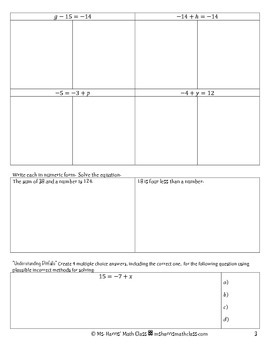# Solving 1-Step Equations Notes Packet (Worksheet)7th - 10th, Homeschool
Subjects
Resource Type
Formats Included
• PDF
Pages
17 pages

### Description

Contents:
-prerequisite review
-practice problems
-exit ticket
-8 student pages
-incorporates writing component for students to write how to solve equations

-subtraction property of equality
-division property of equality
-multiplication property of equality
-negative variables

Common Core Standards:
CCSS.Math.Content.HSA-REI.A.1 Explain each step in solving a simple equation as following from the equality of numbers asserted at the previous step, starting from the assumption that the original equation has a solution. Construct a viable argument to justify a solution method.

CCSS.Math.Content.HSA-REI.A.2 Solve simple rational and radical equations in one variable, and give examples showing how extraneous solutions may arise.

CCSS.Math.Content.HSA-REI.B.3 Solve linear equations and inequalities in one variable, including equations with coefficients represented by letters.

Total Pages
17 pages
Included
Teaching Duration
50 minutes
Report this Resource to TpT
Reported resources will be reviewed by our team. Report this resource to let us know if this resource violates TpT’s content guidelines.